Learn all Concepts of Polynomials Class 9 (with VIDEOS). Check - Polynomials Class 9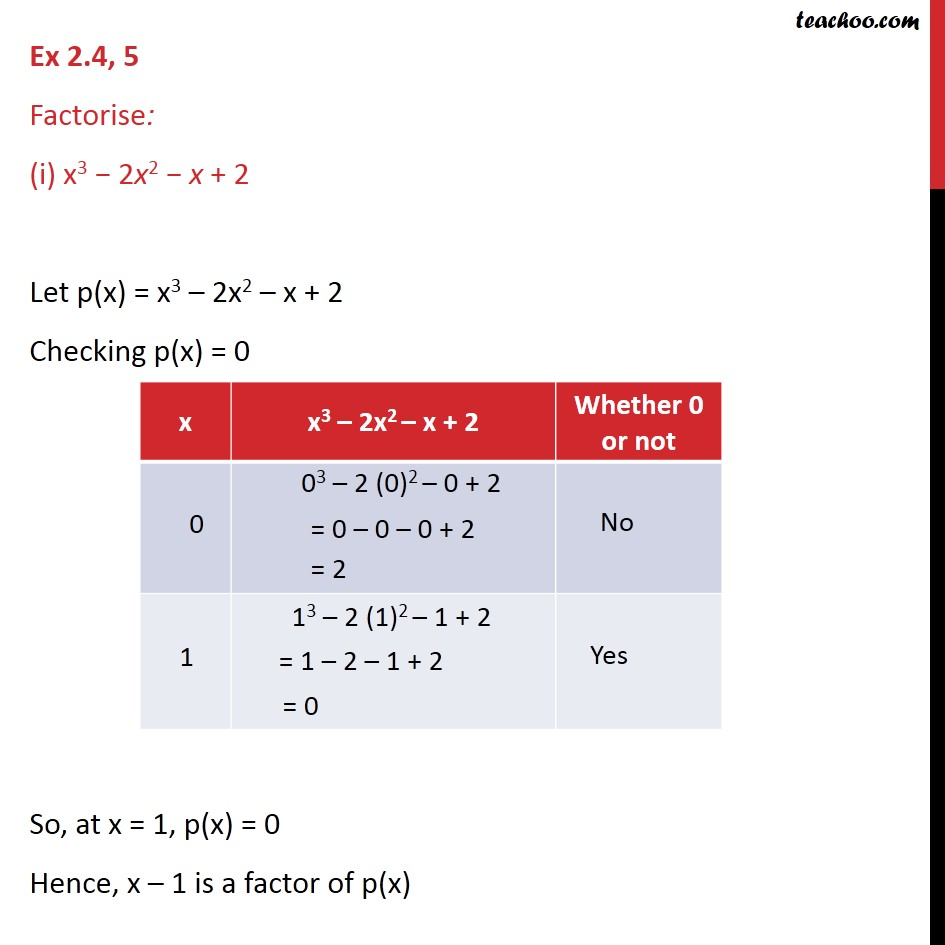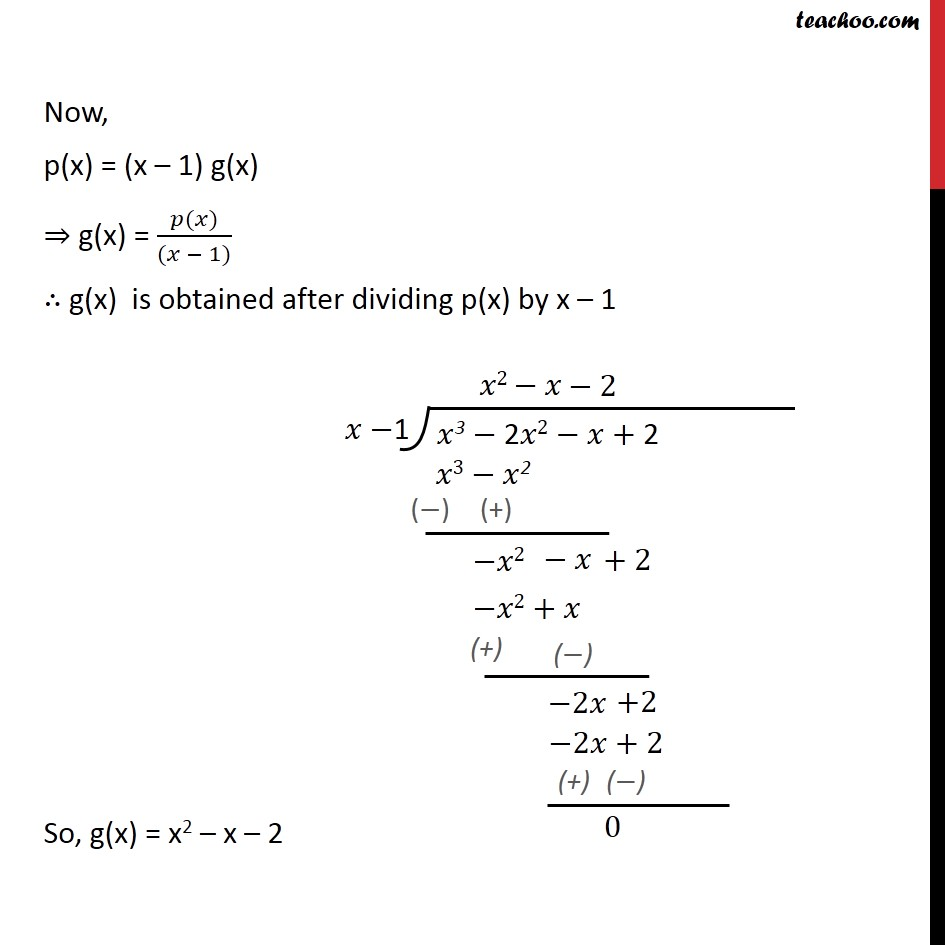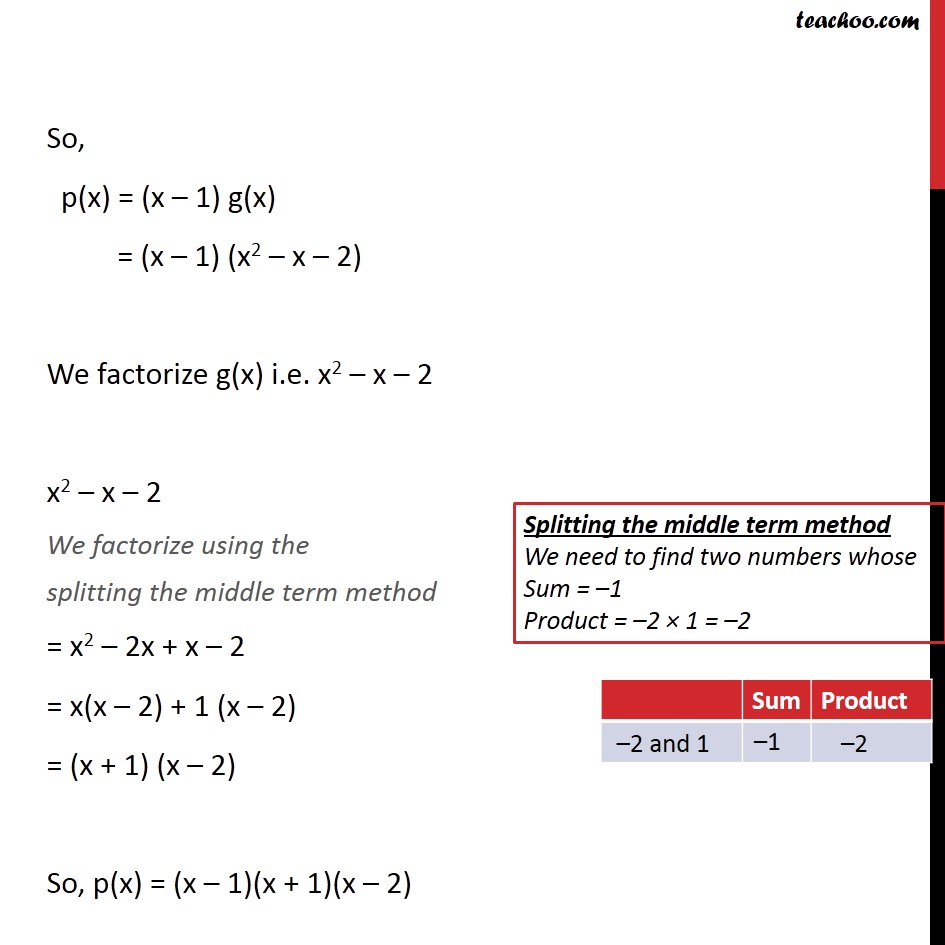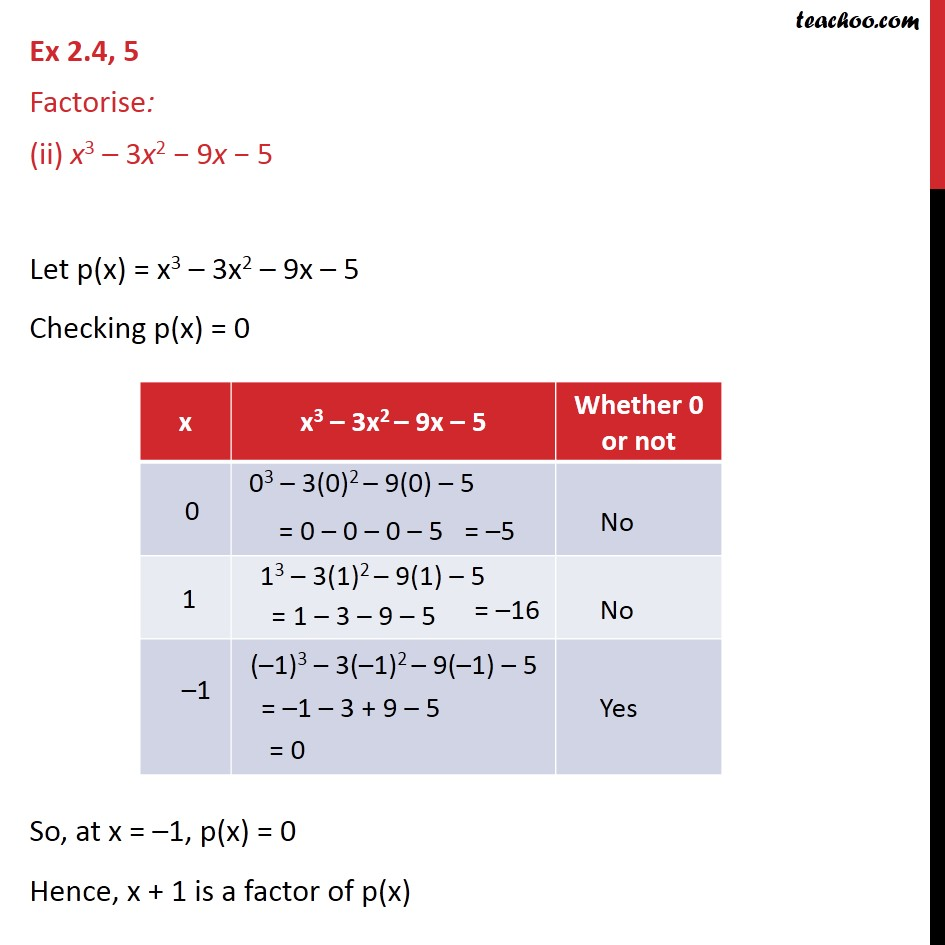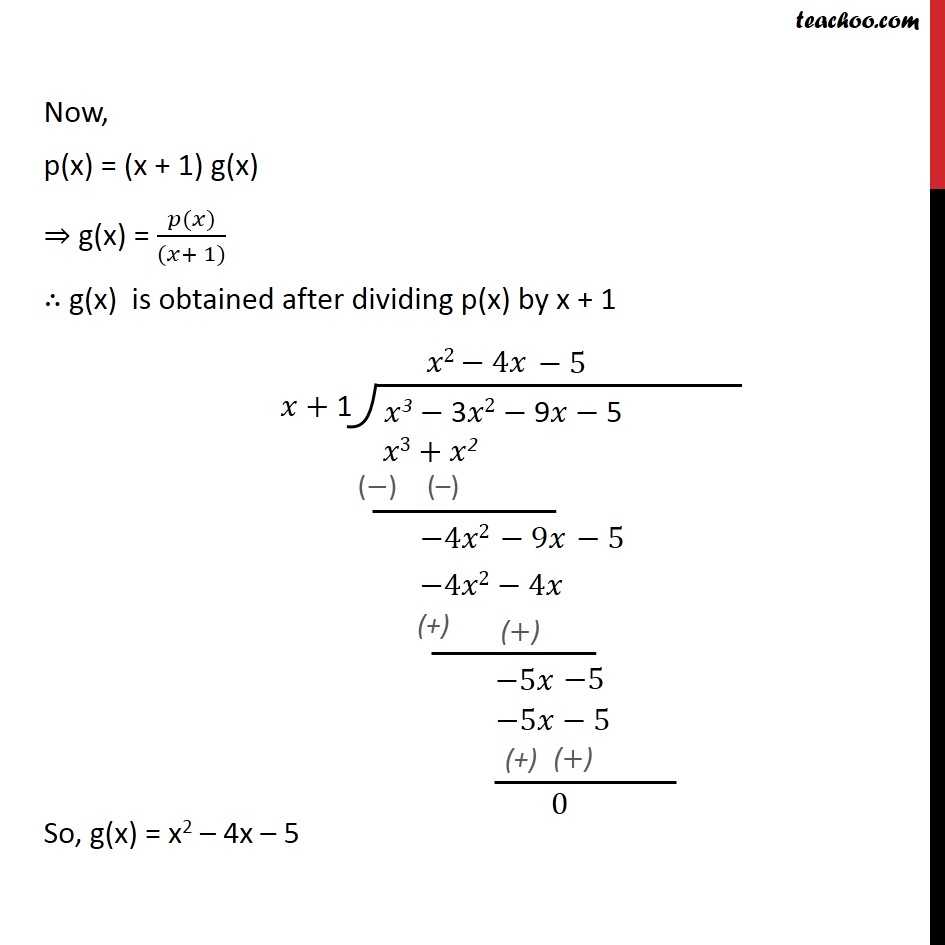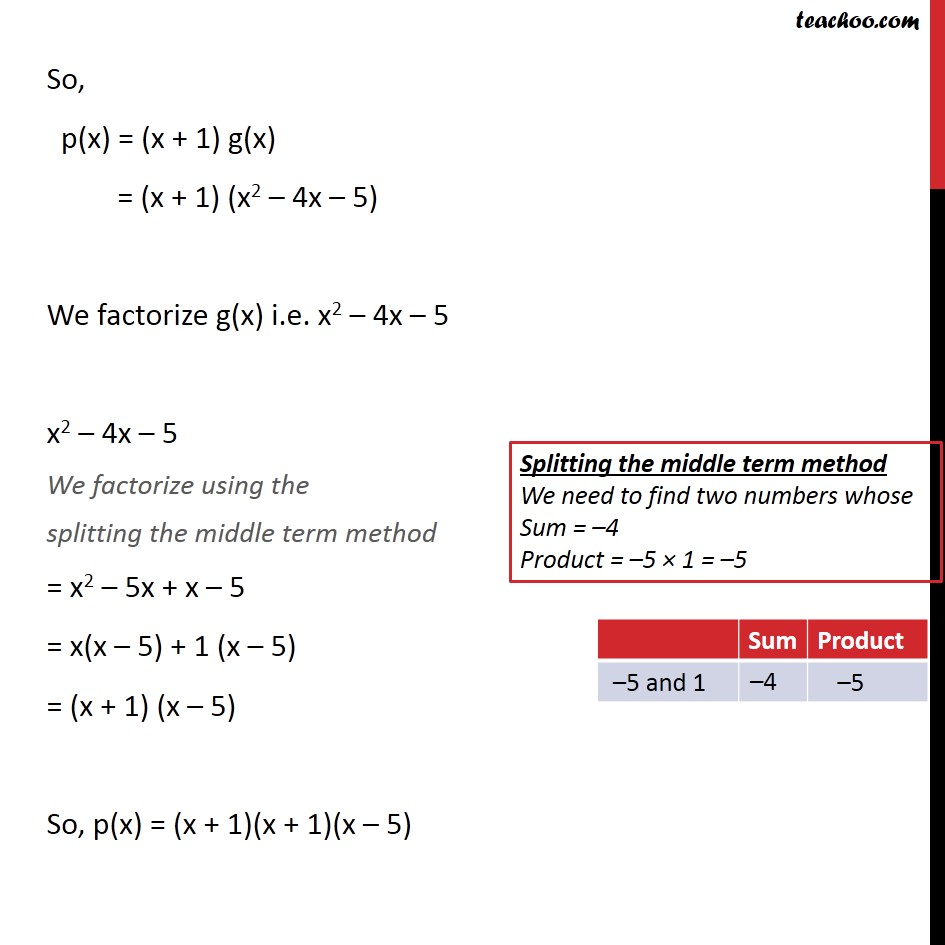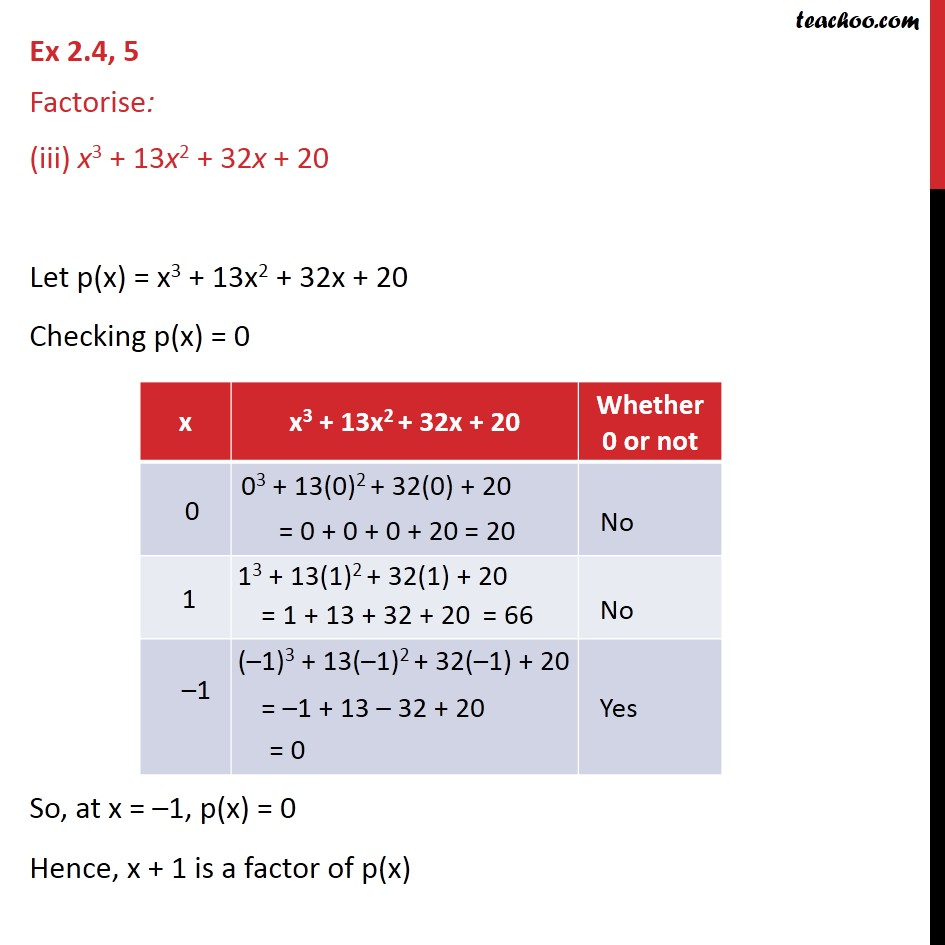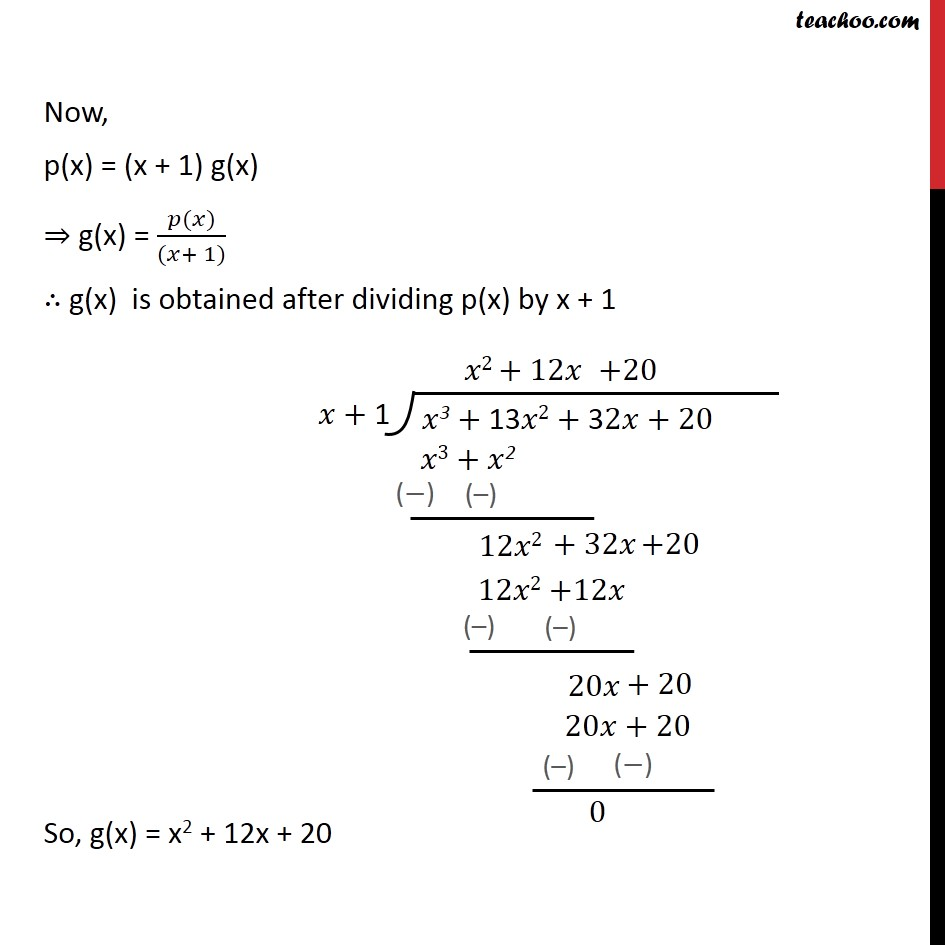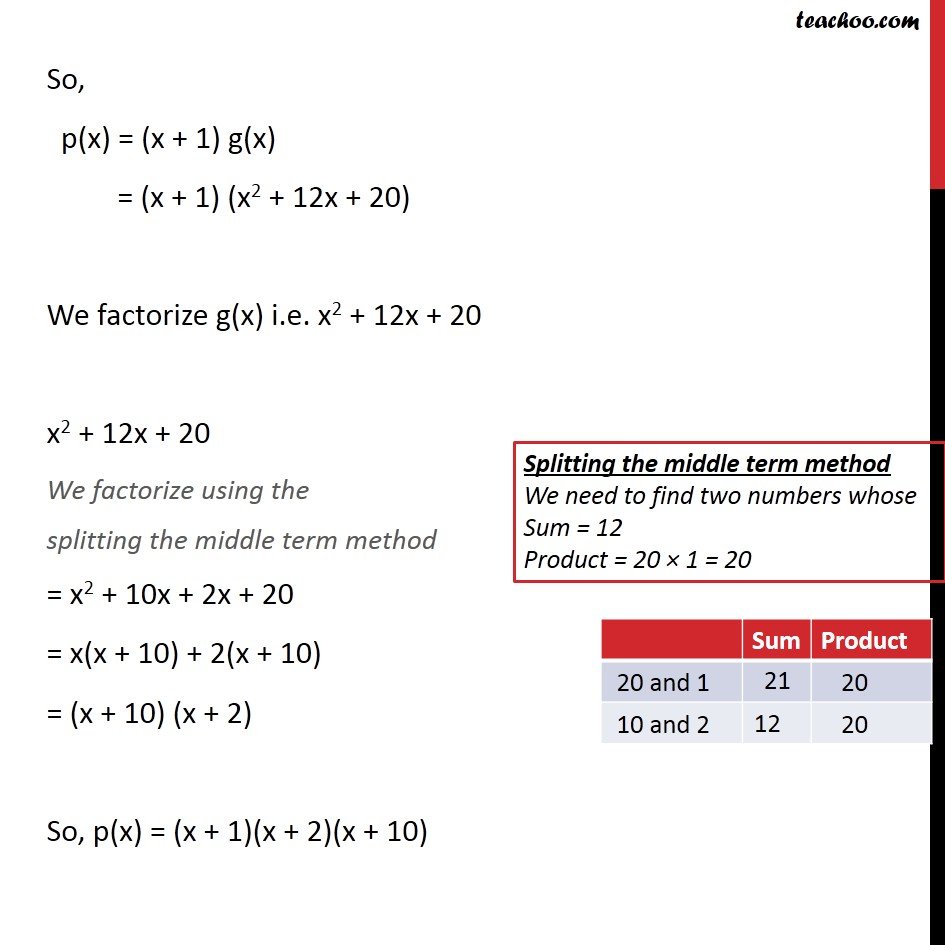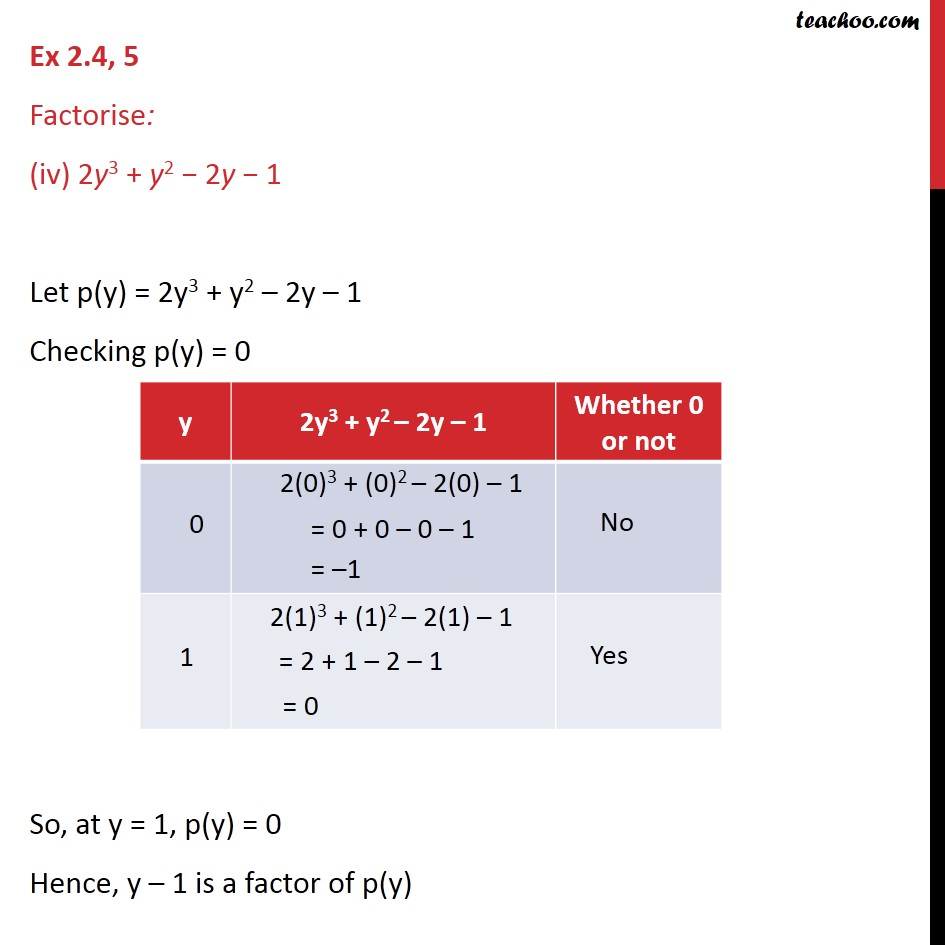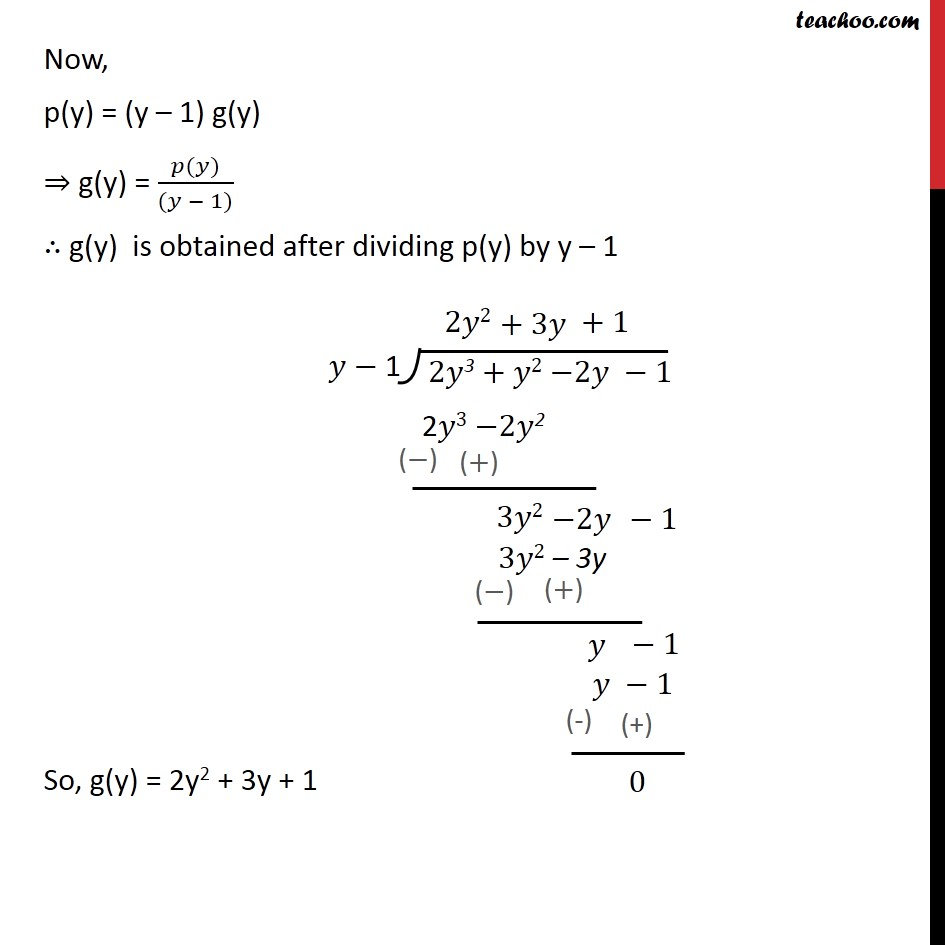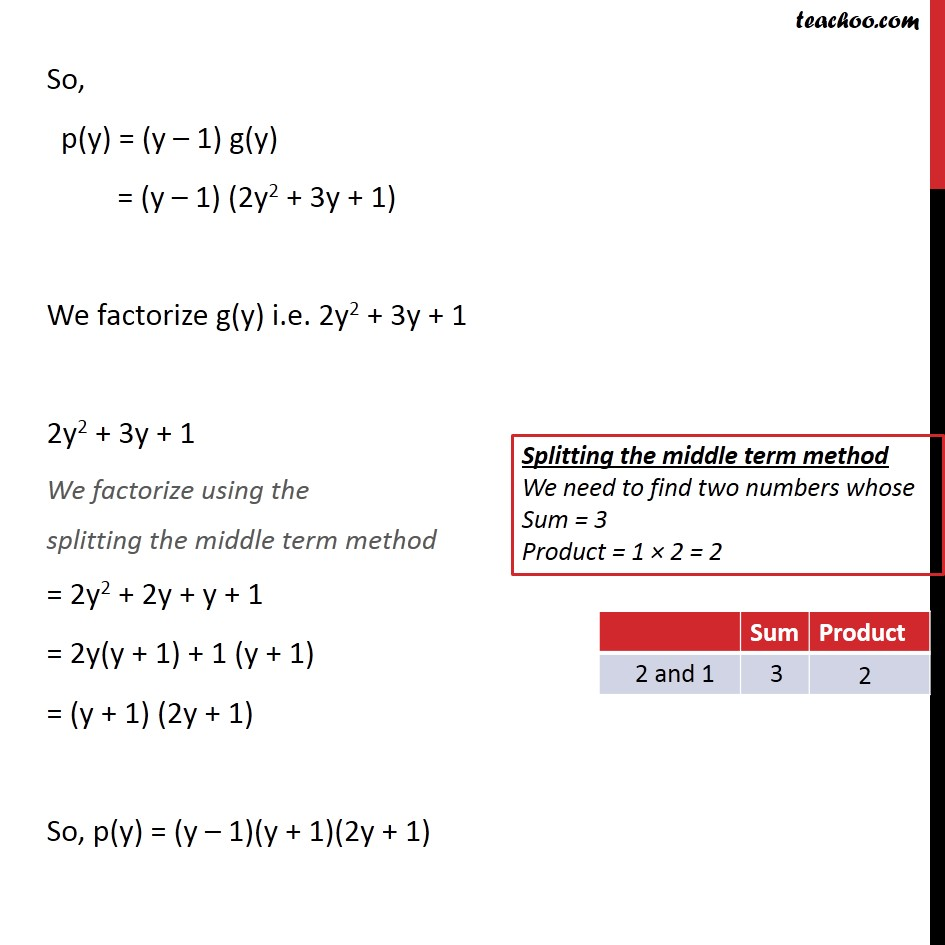1. Chapter 2 Class 9 Polynomials
2. Serial order wise
3. Ex 2.4

Transcript

Ex 2.4, 5 Factorise: (i) x3 − 2x2 − x + 2 Let p(x) = x3 – 2x2 – x + 2 Checking p(x) = 0 So, at x = 1, p(x) = 0 Hence, x – 1 is a factor of p(x) Now, p(x) = (x – 1) g(x) ⇒ g(x) = (𝑝(𝑥))/((𝑥 − 1)) ∴ g(x) is obtained after dividing p(x) by x – 1 So, g(x) = x2 – x – 2 So, p(x) = (x – 1) g(x) = (x – 1) (x2 – x – 2) We factorize g(x) i.e. x2 – x – 2 x2 – x – 2 We factorize using the splitting the middle term method = x2 – 2x + x – 2 = x(x – 2) + 1 (x – 2) = (x + 1) (x – 2) So, p(x) = (x – 1)(x + 1)(x – 2) Ex 2.4, 5 Factorise: (ii) x3 – 3x2 − 9x − 5 Let p(x) = x3 – 3x2 – 9x – 5 Checking p(x) = 0 So, at x = –1, p(x) = 0 Hence, x + 1 is a factor of p(x) Now, p(x) = (x + 1) g(x) ⇒ g(x) = (𝑝(𝑥))/((𝑥+ 1)) ∴ g(x) is obtained after dividing p(x) by x + 1 So, g(x) = x2 – 4x – 5 So, p(x) = (x + 1) g(x) = (x + 1) (x2 – 4x – 5) We factorize g(x) i.e. x2 – 4x – 5 x2 – 4x – 5 We factorize using the splitting the middle term method = x2 – 5x + x – 5 = x(x – 5) + 1 (x – 5) = (x + 1) (x – 5) So, p(x) = (x + 1)(x + 1)(x – 5) Ex 2.4, 5 Factorise: (iii) x3 + 13x2 + 32x + 20 Let p(x) = x3 + 13x2 + 32x + 20 Checking p(x) = 0 So, at x = –1, p(x) = 0 Hence, x + 1 is a factor of p(x) Now, p(x) = (x + 1) g(x) ⇒ g(x) = (𝑝(𝑥))/((𝑥+ 1)) ∴ g(x) is obtained after dividing p(x) by x + 1 So, g(x) = x2 + 12x + 20 So, p(x) = (x + 1) g(x) = (x + 1) (x2 + 12x + 20) We factorize g(x) i.e. x2 + 12x + 20 x2 + 12x + 20 We factorize using the splitting the middle term method = x2 + 10x + 2x + 20 = x(x + 10) + 2(x + 10) = (x + 10) (x + 2) So, p(x) = (x + 1)(x + 2)(x + 10) Ex 2.4, 5 Factorise: (iv) 2y3 + y2 − 2y − 1 Let p(y) = 2y3 + y2 – 2y – 1 Checking p(y) = 0 So, at y = 1, p(y) = 0 Hence, y – 1 is a factor of p(y) Now, p(y) = (y – 1) g(y) ⇒ g(y) = (𝑝(𝑦))/((𝑦 − 1)) ∴ g(y) is obtained after dividing p(y) by y – 1 So, g(y) = 2y2 + 3y + 1 So, p(y) = (y – 1) g(y) = (y – 1) (2y2 + 3y + 1) We factorize g(y) i.e. 2y2 + 3y + 1 2y2 + 3y + 1 We factorize using the splitting the middle term method = 2y2 + 2y + y + 1 = 2y(y + 1) + 1 (y + 1) = (y + 1) (2y + 1) So, p(y) = (y – 1)(y + 1)(2y + 1)

Ex 2.4

Chapter 2 Class 9 Polynomials
Serial order wise